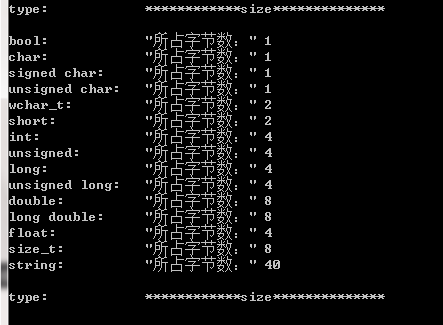• c++数据类型所占字节
千次阅读
2021-07-05 22:38:44

1.整型。
包括：
short（短整型），占2个字节；
int（整型），占4个字节；
long（长整型），占4个字节；
long long（长长整型），占8字节；
整型用来表示一个整数。
2.实型，即浮点型。
包括：
float（单精度浮点型），占4个字节；
double（双精度浮点型），占8个字节；
long double（长精度浮点类型），占8字节；
实型用来表示实数，相对于整型。
3.字符型，即char型。
char（字符型），占1字节；
用来表示各种字符，与ASCII码表一一对应。
4.bool类型
bool（逻辑性变量的定义符），占1个字节c++
更多相关内容
• 关于这个基本的问题，很早以前很清楚了，C标准中并没有具体给出规定那个基本类型应该是多少字节数，而且这个也与机器、OS、编译器有关，比如同样是在32bits的操作系统系，VC++的编译器下int类型4个字节；...
• void DataLength() { qDebug() << “type: \t\t” << “size**” << endl; qDebug() <... QString::fromLocal8Bit(“所占字节数：”) <... QString::fromLocal8Bit(“所占字节数：”) <
void DataLength()
{
qDebug() << "type: \t\t" << "************size**************" << endl;
qDebug() << "bool: \t\t" << QString::fromLocal8Bit("所占字节数：") << sizeof(bool);
qDebug() << "char: \t\t" << QString::fromLocal8Bit("所占字节数：") << sizeof(char);
qDebug()  << "signed char: \t" << QString::fromLocal8Bit("所占字节数：") << sizeof(signed char);
qDebug()  << "unsigned char: \t" << QString::fromLocal8Bit("所占字节数：") << sizeof(unsigned char);
qDebug()  << "wchar_t: \t" << QString::fromLocal8Bit("所占字节数：" )<< sizeof(wchar_t);
qDebug()  << "short: \t\t" << QString::fromLocal8Bit("所占字节数：") << sizeof(short);
qDebug()  << "int: \t\t" << QString::fromLocal8Bit("所占字节数：") << sizeof(int);
qDebug()  << "unsigned: \t" <<QString::fromLocal8Bit( "所占字节数：") << sizeof(unsigned);
qDebug()  << "long: \t\t" << QString::fromLocal8Bit("所占字节数：" )<< sizeof(long);
qDebug()  << "unsigned long: \t" <<QString::fromLocal8Bit( "所占字节数：" )<< sizeof(unsigned long);
qDebug()  << "double: \t" << QString::fromLocal8Bit("所占字节数：") << sizeof(double);
qDebug()  << "long double: \t" << QString::fromLocal8Bit("所占字节数：") << sizeof(long double);
qDebug()  << "float: \t\t" << QString::fromLocal8Bit("所占字节数：" )<< sizeof(float);
qDebug()  << "size_t: \t" << QString::fromLocal8Bit("所占字节数：") << sizeof(size_t);
qDebug()  << "string: \t" << QString::fromLocal8Bit("所占字节数：") << sizeof(string) << endl;
qDebug()  << "type: \t\t" << "************size**************" << endl;
}cout << "type: \t\t" << "************size**************" << endl;
cout << "bool: \t\t" << "所占字节数：" << sizeof(bool);
cout << "\t最大值：" << (numeric_limits<bool>::max)();
cout << "\t\t最小值：" << (numeric_limits<bool>::min)() << endl;
cout << "char: \t\t" << "所占字节数：" << sizeof(char);
cout << "\t最大值：" << (numeric_limits<char>::max)();
cout << "\t\t最小值：" << (numeric_limits<char>::min)() << endl;
cout << "signed char: \t" << "所占字节数：" << sizeof(signed char);
cout << "\t最大值：" << (numeric_limits<signed char>::max)();
cout << "\t\t最小值：" << (numeric_limits<signed char>::min)() << endl;
cout << "unsigned char: \t" << "所占字节数：" << sizeof(unsigned char);
cout << "\t最大值：" << (numeric_limits<unsigned char>::max)();
cout << "\t\t最小值：" << (numeric_limits<unsigned char>::min)() << endl;
cout << "wchar_t: \t" << "所占字节数：" << sizeof(wchar_t);
cout << "\t最大值：" << (numeric_limits<wchar_t>::max)();
cout << "\t\t最小值：" << (numeric_limits<wchar_t>::min)() << endl;
cout << "short: \t\t" << "所占字节数：" << sizeof(short);
cout << "\t最大值：" << (numeric_limits<short>::max)();
cout << "\t\t最小值：" << (numeric_limits<short>::min)() << endl;
cout << "int: \t\t" << "所占字节数：" << sizeof(int);
cout << "\t最大值：" << (numeric_limits<int>::max)();
cout << "\t最小值：" << (numeric_limits<int>::min)() << endl;
cout << "unsigned: \t" << "所占字节数：" << sizeof(unsigned);
cout << "\t最大值：" << (numeric_limits<unsigned>::max)();
cout << "\t最小值：" << (numeric_limits<unsigned>::min)() << endl;
cout << "long: \t\t" << "所占字节数：" << sizeof(long);
cout << "\t最大值：" << (numeric_limits<long>::max)();
cout << "\t最小值：" << (numeric_limits<long>::min)() << endl;
cout << "unsigned long: \t" << "所占字节数：" << sizeof(unsigned long);
cout << "\t最大值：" << (numeric_limits<unsigned long>::max)();
cout << "\t最小值：" << (numeric_limits<unsigned long>::min)() << endl;
cout << "double: \t" << "所占字节数：" << sizeof(double);
cout << "\t最大值：" << (numeric_limits<double>::max)();
cout << "\t最小值：" << (numeric_limits<double>::min)() << endl;
cout << "long double: \t" << "所占字节数：" << sizeof(long double);
cout << "\t最大值：" << (numeric_limits<long double>::max)();
cout << "\t最小值：" << (numeric_limits<long double>::min)() << endl;
cout << "float: \t\t" << "所占字节数：" << sizeof(float);
cout << "\t最大值：" << (numeric_limits<float>::max)();
cout << "\t最小值：" << (numeric_limits<float>::min)() << endl;
cout << "size_t: \t" << "所占字节数：" << sizeof(size_t);
cout << "\t最大值：" << (numeric_limits<size_t>::max)();
cout << "\t最小值：" << (numeric_limits<size_t>::min)() << endl;
cout << "string: \t" << "所占字节数：" << sizeof(string) << endl;
// << "\t最大值：" << (numeric_limits<string>::max)() << "\t最小值：" << (numeric_limits<string>::min)() << endl;
cout << "type: \t\t" << "************size**************" << endl;
system("pause");

展开全文• 本篇文章主要是对C/C++与Java各数据类型所占字节数进行了详细的对比。需要的朋友可以过来参考下，希望对大家有所帮助
• C标准中并没有具体给出规定那个基本类型应该是多少字节数，具体与机器、OS、编译器有关，比如同样是在32bits的操作系统系，VC++的编译器下int类型4个字节；而tuborC下则是2个字节。 所以int，long int，...

C标准中并没有具体给出规定那个基本类型应该是多少字节数，具体与机器、OS、编译器有关，比如同样是在32bits的操作系统系，VC++的编译器下int类型为占4个字节；而tuborC下则是2个字节。

所以int，long int，short int的宽度都可能随编译器而异。但有几条铁定的原则（ANSI/ISO制订的）：

• sizeof(short int)<=sizeof(int)

• sizeof(int)<=sizeof(long int)

• short int至少应为16位（2字节）

• long int至少应为32位。

下面给出不同位数编译器下的基本数据类型所占的字节数：

16位编译器

char ：1个字节
char*(即指针变量): 2个字节
short int : 2个字节
int：  2个字节
unsigned int : 2个字节
float:  4个字节
double:   8个字节
long:   4个字节
long long:  8个字节
unsigned long:  4个字节

32位编译器

char ：1个字节
char*（即指针变量）: 4个字节（32位的寻址空间是2^32, 即32个bit，也就是4个字节。同理64位编译器）
short int : 2个字节
int：  4个字节
unsigned int : 4个字节
float:  4个字节
double:   8个字节
long:   4个字节
long long:  8个字节
unsigned long:  4个字节

64位编译器

char ：1个字节
char*(即指针变量): 8个字节
short int : 2个字节
int：  4个字节
unsigned int : 4个字节
float:  4个字节
double:   8个字节
long:   8个字节
long long:  8个字节

unsigned long:  8个字节

下页是一个简单的测试例子(C++)：

运行环境，Windows 7旗舰版 + vc++6.0 + 32位系统

#include<iostream>
using namespace std;

void main()
{
cout<<sizeof(char)<<endl;  //1
cout<<sizeof(short)<<endl;  //2
cout<<sizeof(int)<<endl;  //4
cout<<sizeof(long)<<endl;  //4
cout<<sizeof(float)<<endl;  //4
cout<<sizeof(double)<<endl;  //8

cout<<sizeof(char *)<<endl;  //4
cout<<sizeof(short *)<<endl; //4
cout<<sizeof(int *)<<endl;  //4
cout<<sizeof(long *)<<endl;  //4
cout<<sizeof(float *)<<endl; //4
cout<<sizeof(double *)<<endl; //4

//cout<<sizeof(void)<<endl;  //这句无法通过编译，可能是因为void代表空，不包含任何东西
cout<<sizeof(void *)<<endl;  //4(相对于上一句，这句挺好理解的，"void *" 是一个指针)
}

展开全文数据类型 int
• 与OS、编译器有关，比如同样是在32位操作系统，VC++的编译器下int类型为4个字节，而在tuborC下则是2个字节。 #include<iostream> using namespace std; int main() { cout << "int类型占用的字节数：" ...

OS编译器有关，比如同样是在32位操作系统，VC++的编译器下int类型为4个字节，而在tuborC下则是2个字节。

#include<iostream>
using namespace std;
int main()
{
cout << "int类型占用的字节数：" << sizeof(int) << endl;
cout << "short int类型占用的字节数：" << sizeof(short int) << endl;
cout << "unsigned int类型占用的字节数：" << sizeof(unsigned int) << endl;
cout << "long int类型占用的字节数：" << sizeof(long) << endl;
cout << "long long类型占用的字节数：" << sizeof(long long) << endl;
cout << "float类型占用的字节数：" << sizeof(float) << endl;
cout << "double类型占用的字节数：" << sizeof(double) << endl;
cout << endl << "下面是指针类型！" << endl;
cout << "int*类型占用的字节数：" << sizeof(int*) << endl;
cout << "short int*类型占用的字节数：" << sizeof(short int*) << endl;
cout << "long int*类型占用的字节数：" << sizeof(long*) << endl;
cout << "double*类型占用的字节数：" << sizeof(double*) << endl;
cout << "char*类型占用的字节数：" << sizeof(char*) << endl;
}


我在VS2017下运行的结果如下：long long 占用8个字节。 8*8=64bit。最大表示数为2^63。最多19位。
可以得到指针类型都占8个字节。

展开全文• 1.基本数据类型 char : 1 short: 2 int: 4 long long: 8 float:4 double:8 bool:1 2. 指针与引用 3.构造类型 3.1 struct 由于struct有**边界对齐(凑4或8)**的要求 所以尽量将同类型的写一块 类型小的放在最前面 关于...c++
• 了解内存模型可以把指针用得炉火纯青，各种对内存基本单元——字节（byte） 随意操作 1 内存 1.1 内存、内存地址、地址空间 1.1.1 内存 计算机的内存是一块用于存储数据的空间，由一系列连续的存储单元组成，每个...
• 常见变量类型常见的变量类型有char、short、int、long、float、double，与之相对应的还有几个无符号的类型，只需要前面加上unsigned即可，例如unsigned char、unsigned int等，另外还有些typedef出来的类型例如WORD...编译器 C++
• 在vs 2017环境中使用sizeof了解各个数据类型的大小 整形类型 short、int、long、long long #include <iostream> using namespace std; int main() { cout << "short 类型所占内存空间为： " << ...c++
•  C跟java的基本数据所占位数的差异，比如32位系统，java long型多少位,c的long型多少位,64位呢？所以在这里把相关涉及的知识整理总结一下，防止遗忘。   C/C++ :  1字节=8位  1k=1024字节=2^10  1m=...java 基本数据类型
• 1、整形：int、long 2、字符型：char、wchar_t 3、布尔型：bool 4、浮点型：float、double 5、指针 ...　int占据操作系统一个内存单元的大小。...64位操作系统一个内存单元是16位，故8个字节。 ...
• 注：每种数据类型的取值范围都是与编译器相关的，以下为数据Visual C++32位环境下的参数，如想清楚了解自己所用编译器对各数据类型所占字节，可以用sizeof进行检验。也可以使用limits.h头文件中的INT_MIN,INT_MAX等...数据类型
• 二、不同位数编译器下的基本数据类型所占字节数 1、16位编译器 2、32位编译器 3、64位编译器 三、拓展小结 叮嘟！这里是小啊呜的学习课程资料整理。好记性不如烂笔头，今天也是努力进步的一天。一起加油进阶吧！c++ 编程语言 c语言 编译器
• 基本数据类型占用的字节数 32位编译器： char ：1个字节 char*（即指针变量）: 4个字节（32位的寻址空间是2^32, 即32个bit，也就是4个字节） short int : 2个字节 int： 4个字节 unsigned int : 4个字节 ...字节数 C++
• 1.C++数据类型简介 C++是一种强类型语言，任何变量或函数必须遵循“先申明后使用”的原则。定义数据类型有两个方面的作用：一是决定该类型的数据在内存中如何存储，二是决定可对该类型的数据进行哪些合法的运算。 ...
• 基本数据类型所占字节大小： 32位编译器（指针4字节） char ：1个字节 short int :4 字节 int： 4个字节 long: 4个字节 double: 8个字节 long long: 8个字节 64位编译器（指针8字节） char ：1个字节 ...sizeof c++
• 转自： ...  和机器字长及编译器有关系： 所以，int，long int，short int的宽度...虚函数因为存在一个虚函数表，需要4个字节数据成员对象如果为指针则为4字节，注意有字节对齐，如果为13字节，则进位到16字节空间。
• C语言入门经典——基础知识（数据类型）：https://blog.csdn.net/weixin_42167759/article/details/80404815 闲聊c/c++: 各平台下基本数据类型字节长度：https://www.jianshu.com/p/1116...
• char*（即指针变量）: 4个字节（32位的寻址空间是2^32, 即32个bit，也就是4个字节。同理64位编译器） short int : 2个字节 int： 4个字节 unsigned int : 4个字节 float: 4个字节 double: 8个字节 long: 4个字节...C++ c语言
• // c++类型所占字节数查看方式，可以输出每个类型数据占用几字节数据
• 在C/C++中基本数据类型因开发环境的不同而不同。但是，C标准中并没有具体...下面给出不同位数编译器下的基本数据类型所占字节数： 在Java中，所有的数值类型占据的字节数量与平台无关！ 并且在Java中没有任何无基本数据类型
• 准备校招笔试的时候经常遇到C++某个数据类型占据多少个字节的问题，查阅了下资料，总结如下： 首先罗列一下C++中的数据类型都有哪些： 1、整形：int、long、short(2字节) 2、字符型：char、wchar_t 3、布尔型...
• 各个类型的变量长度由编译器来决定（实际上与操作系统位数和编译器都有关）使用时可用sizeof（）得到，当前主流编译器一般是32位或64位。　类型　16位 32位　64位　char　1 1　1　short int　2 2　2　int　2 4　...
• 1.各个数据类型占用字节大小 #include<iostream> using namespace std; int main(){ cout<<sizeof(char)<<endl; //1个字节，-128~+127 -2^7~2^7-1 cout<<sizeof(short)<<endl...
• 作者：billy 版权声明：著作权归作者所有，商业转载请联系作者获得授权，非商业转载请注明出处 前言 在考虑内存管理之前，我们要先...博主在这里整理了一些基础元素所占字节大小，防止长时间不接触而遗忘掉。 ......

# c++数据类型所占字节c++ 订阅